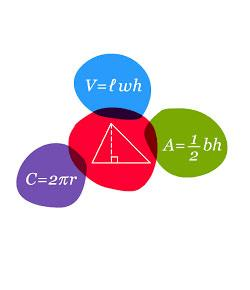# Algebra I Probability Quiz

3 Questions | Total Attempts: 4229SettingsThis is a quiz for review of probability for Algebra I students. There will be five questions. For proficiency each student must accomplish an 75% on the quiz.

• 1.
If George is rolling one die, what is the probability that he will roll a 6?
• A.

6

• B.

1/6

• C.

1/3

• D.

6/6

• E.

None of the above

• 2.
If a bag contains 3 white marbles, 4 red marbles, and 5 blue marbles, what is the probability of selecting a red or a blue marble?
• A.

8

• B.

3/4

• C.

1/4

• D.

1/9

• E.

None of the above

• 3.
If a bag contains 3 white marbles, 4 red marbles, and 5 blue marbles, what is the probability of selecting a red marble and then selecting a blue marble without replacement?
• A.

5/33

• B.

7/60

• C.

5/132

• D.

7/132

• E.

None of the above

Related Topics